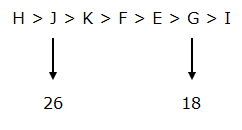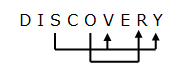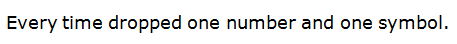# RRB PO Prelims Reasoning (Day-21)

## Reasoning Materials for RRB PO Prelims

Dear Aspirants, Our IBPS Guide team is providing new series of Reasoning Questions for RRB PO Prelims 2020 so the aspirants can practice it on a daily basis. These questions are framed by our skilled experts after understanding your needs thoroughly. Aspirants can practice these new series questions daily to familiarize with the exact exam pattern and make your preparation effective.

Start Quiz

IBPS RRB PO Prelims

Order and ranking

Direction (1-4): Answer the question based on the information given below.

Seven students viz. E, F, G, H, I, J and K are having different number of chocolates with them, but not necessarily in the same order. E has less number of chocolates than K but more number of chocolates than G. J has more number of chocolates than F. Not more than two persons has less number of chocolate than E. F has less number of chocolates than K but more than E.H has more number of chocolates than J. I doesn’t have more number of chocolates than G. The person with the second least number of chocolates has 18 chocolates whereas the person with the second highest number of chocolates has 26 chocolates. H does not have 26 chocolates.

1) Who among the following person has the third highest number of chocolates?

A. J

B. I

C. F

D. K

E. None of these

2) How many possible chocolates does H has?

A.26

B.32

C.18

D.12

E. None of these

3) Who among the following person has second highest chocolates than G?

A.F

B.E

C.K

D.J

E. None of these

4) How many persons have less number of chocolates than J?

A. One

B. Four

C. Five

D. Three

E. None of these

Alphabet series

Direction (5-8): Answer the question based on the information given below.

TIP    BOT  CUP   SKY   DAY   NET

5) If all the words are arranged in dictionary order from right to left, then how many words remain unchanged in their position?

A. One

B. Two

C. Three

D. More than three

E. None

6) How many letters are there accordingto the English alphabetical series between the third letter of the first word from the left and the first letter of the second word from the right end?

A. Ten

B. Twelve

C. Eleven

D. Nine

E. More than twelve

7) If all the letters are arranged in alphabetical order from left to right within the word and then arranged in dictionary order from right to left, then which of the following letter is the second letter of the third word from the left?

A.E

B.N

C.P

D.T

E.None of these

8) If all the letters are reversed within the word, then how many words thus formed are meaningful?

A. Three

B. More than three

C. None

D. Two

E. One

Misc

9) How many such pairs of letters are there in the word “DISCOVERY” which have as many letters between them as there are in the English alphabets (both forward and backward)?

A. One

B. Two

C. Three

D. More than three

E. None

10) What will come in place of (?).

54321%#\$&5432%#\$543%#?

A. \$54

B.54%

C.43%

D.543

E.3%#

Directions (1-4):

From the given information, the following arrangements were made.Directions (5-8) :

TIP    BOT  CUP   SKY   DAY   NET

TIP   SKY   NET   DAY   CUP   BOT

Hence, option A is correct.

TIP   BOT  CUP   SKY   DAY  NET

DE F G H I J K L M N O P

Hence, option C is correct.

TIP    BOT  CUP   SKY   DAY   NET

IPT    BOT  CPU   KSY   ADY   ENT

KSY   IPT    ENT  CPU   BOT  ADY

Hence, option B is correct.

TIP    BOT  CUP   SKY   DAY   NET

PIT   TOB  PUC   YKS   YAD   TEN

Hence, option D is correct.Hence, option D is correct.